# Unequal, N-way Gysel power divider

Click here to go to our main page on Gysel power dividers

Click here to see a three-way rat race (Gysel) planar power divider (new for October 2017)

New for August 2016!

This page was created from content submitted by Richard from the Down Under, who has helped us out in the past.  What if you wanted to make an unequal-split Gysel power divider? What are the constraints?

Below is a the simplest schematic of a four-way Gysel, where we have parameterized the left and right transmission line sections so that they can have unequal impedances.  The middle sections are all the same (more about that later). Even though this is a four-way (five-port) circuit, anyone with the slightest math skills could scale it to three-way and four-way, so don't say we misled you with the title of this page referring to "N-way"...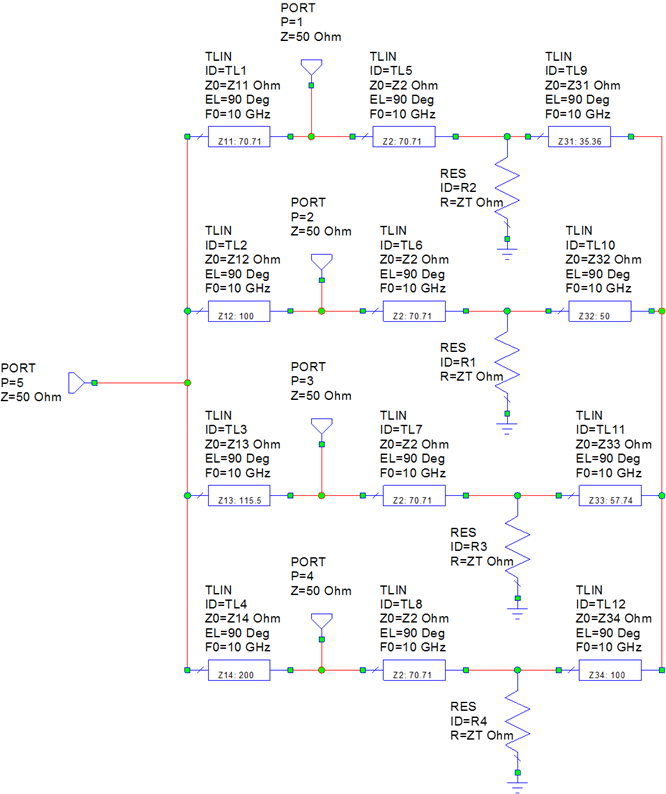Somewhere below are equations to set up the twelve section impedances.  The "ratios" are the power from the common port to each divider port.  Needless to say, the four fractions have to add up to unity, or the math falls apart.  There are many ways to specify the power at each port, you could come up with equations where you specify the dB differences between three of the ports to a reference port.  Knock yourself out!

Z0 was chosen as 50 ohms but we left it in the equations so you could design in different impedances.  ZT is the termination impedances and is a degree of freedom; we chose 100 ohms; it will affect the bandwidth of some parameters so be sure to try different values.

The Z11, Z12, Z13 and Z14 impedances are functions of the power fraction to each port.

The four Z2 impedance segments depends only on your choice of ZT termination impedance.  As shown, all the split ports will be matched.

Impedances Z31, Z32, Z33 and Z34 are also a degree of freedom.  We set them all to a fraction of Z0 we called ZF.  Typically low impedances are used in these legs, on our case they are 25 ohms.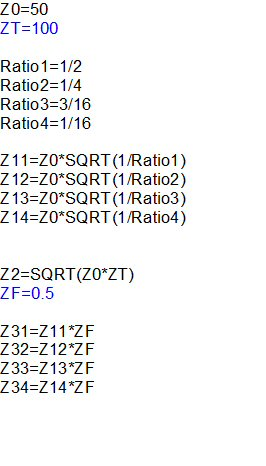Now let's look at the predicted performance. First we will look at the loss.  Port 2 indeed has 1/2 the power at the combined port, or -3.01 dB. Port 2 has 1/4 the power (-6.02 dB), port 3 has 3/16 (-7.27 dB) and port 4 has 1/16 of the power (-12.04 dB), go ahead and convert the decibel values to verify that if you are skeptical.  Fidete, sed verificate!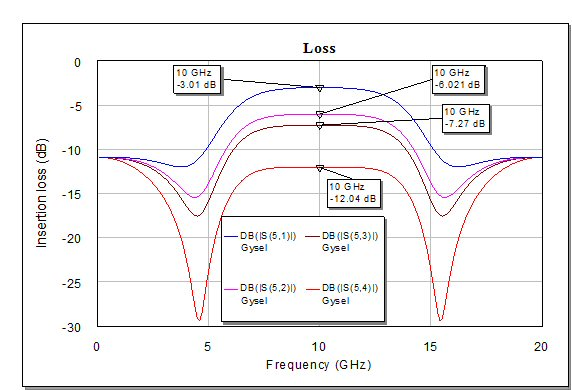Below are the return losses, perfect at the center frequency.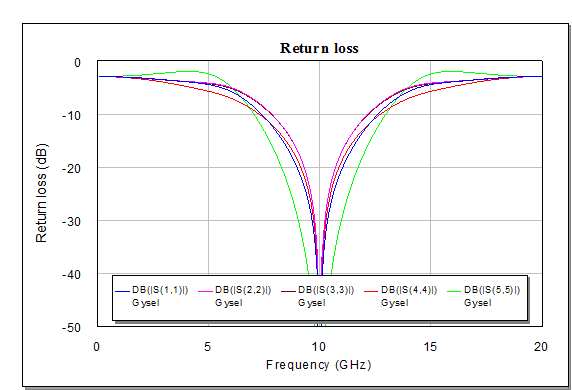And here are four of the isolations, also perfect at center frequency. Note that there are are really six terms, as S13 and S42 will be different. Don't be lazy like us, plot them yourself.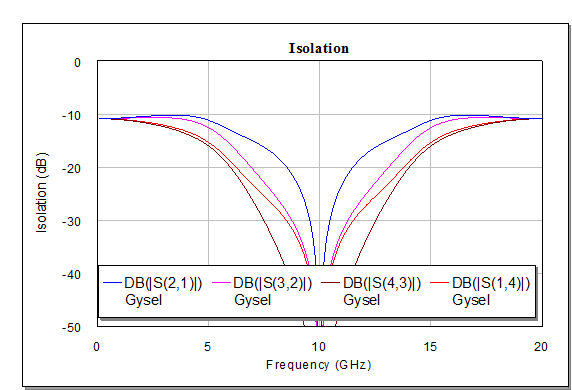So, what about the practical limits of this network? Go back to the schematic and see that we included the calculated impedances in for all sections. The ports with low coupling values are forced to have high impedances, in this case Z14 has 200 ohms impedance.  Such a high value is not possible.  In practice, you might consider using this technique only if the coupling values are all within a few dB of each other, like power fractions of 3/10, 3/10, 2/10 and 2/10. On the other hand, as Richard points out, at low frequencies you can approximate high-impedance lines with lumped elements.

Author : Unknown Editor